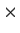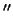Next: *rosprf* - Using the Up: Source Subtraction Previous: Source Subtraction

### *immodel* - subtracting a King model

In the following example we use a file which is scheduled for release in the archive at the end of 1994 (rh800021). Although it is possible to make subtractions on photon arrays, we have chosen to first smooth the data because it is easier to evaluate the residuals and intervening steps.

We use imsmooth (section 6.4) to extract a 512512 array at full pixel resolution (0.5) with a minimal (compared to the HRI PRF) Gaussian smoothing function; sigma = 4.24 p, producing 5FWHM. We then evaluate this image, determining the center of the cluster gas distribution and measure the total number of counts in order to assign an intensity to the model. NB: imsmooth conserves counts.

Running immodel, we provide the following parameters:

input 	the array created by imsmooth
func	King
arg1	70	this is in pixels, so the core radius is 35"
arg2	1.75	for a beta value of 3/4, the exponent is 1.75
normalize yes
scale	-1	this is a global scaling; negative sign means subtract.
sources 257,256,5000	pixel coordinates and amplitude.


The normalization of intensity is somewhat obscure. The 5000 number is not counts; see below. The residual map can be examined with imcontour, tvproj, and the like to see if the model parameters need changing.

Another way to accomplish the same result is afforded by changing the input specification to 512 512" and the scale parameter to +1. This creates an output file with only the model present, thereby allowing one to measure the effective number of counts in the model with imcnts. In our example we specified 5000 as the intensity of the model. Measuring the effective counts in a circle with r=250 pix (i.e. almost the entire array), we find a value of 9342. Hence the term obscure" used earlier. Since our data had approximately 10,700 counts in the same circle, we used a scaling factor (SF=1.145) with imcalc in order to minimize the residual:

imcalc
input	RESIDUAL = SMOOTHED_DATA - SF * MODEL


The help file for immodel lists other functions available as well as options for user supplied functions.Next: *rosprf* - Using the Up: Source Subtraction Previous: Source Subtraction
rsdc@cfa.harvard.edu
1998-06-10